## Modeling Gravity

### Gravity Models

Gravity influences motion in many natural and engineered systems. These range in scale from the very large, such as the planets orbiting the sun, to the relatively small, such as the shock absorbers damping gravity-driven oscillations in a car. In Simscape™ Multibody™, you can add gravity to systems like these using three gravity models:

• Uniform gravity, as experienced by most earthbound systems. The force on each body due to uniform gravity depends only on its mass. This force is the same everywhere in space for a given body, though it can vary in time. You model uniform gravity using the Mechanism Configuration block.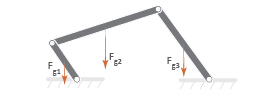• Gravitational field, as experienced by the planets in the solar system. The force on each body due to a gravitational field depends not only on its mass but also on its inverse square distance to the field origin. You model a gravitational field using the Gravitational Field block.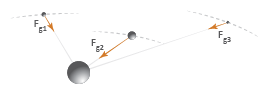• Inverse-square law force pair, similar in nature to a gravitational field, but acting exclusively between one pair of bodies. You model an inverse-square law force pair using the Inverse Square Law Force block. You must specify the body masses and force constants explicitly.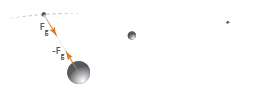### Gravitational Force Magnitude

The force of gravity is an inverse-square law force—that is, one that decays with the square distance from the field origin to the target body. The magnitude of this force, Fg, follow from Newton’s law of universal gravitation which, for two bodies of mass M and m a distance R apart, states

`${F}_{g}=-G\frac{Mm}{{R}^{2}}$`

with G being the gravitational constant. This is the force that you model when you represent gravity through Gravitational Field or Inverse Square Law Force blocks. If the distance between source and target masses is constant, the gravitational force reduces to a simpler form,

`${F}_{g}=-mg$`

with g being the nominal gravitational acceleration. Near the surface of the Earth, at a distance equal to Earth’s radius from the gravitational field origin, the nominal acceleration equals

`$g=\frac{GM}{{R}^{2}}\approx 9.80665\text{\hspace{0.17em}}m/{s}^{2}$`

This is the gravitational force that you model when you represent gravity through the Mechanism Configuration block. The figure shows how the magnitude of the gravitational force (Fg) varies with distance (R) for a given body under uniform gravity, a gravitational field, and an inverse-square law force pair.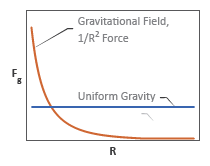### Force Position and Direction

In a physical system, the force due to a gravitational field acts at a body’s center of mass—automatically computed during simulation—along the imaginary line connecting the field origin to the center of mass. These are also the application point and direction of gravity that the Gravitational Field block provides. See Modeling Bodies for more information on how Simscape Multibody defines a body subsystem.

Far from the field origin, the field origin-center of mass line remains approximately constant at small-to-moderate displacements, and the force of gravity behaves as if its direction were fixed. This is the approximation used in the Mechanism Configuration block. Gravity still acts at each body’s center of mass, but its direction is now fixed along the gravity vector that you specify.

If you want to model the effects of gravity on a point other than a body’s center of mass, you can add a frame at the desired location and apply a gravitational force directly at that frame. You model the force using the Inverse Square Law Force block. This force points along the imaginary line between the two body frames that the Inverse Square Law Force block connects.

The table summarizes the application point and direction of gravity provided by the different blocks.

BlockPositionDirection
Mechanism ConfigurationCenter of MassSpecified gravity vector
Gravitational FieldCenter of MassField origin-center of mass line
Inverse Square Law ForceConnection framesBase-follower frame line

### Gravitational Torques

A gravitational torque can arise in a large body immersed in a nonuniform gravitational field. The lemon-shaped moon, with its near end perpetually facing Earth, is one example. Being placed at different distances from Earth, the near and far elongated ends experience dissimilar gravitational forces, resulting in a net gravitational torque if the line between the two ends ever falls out of alignment with the center of the Earth.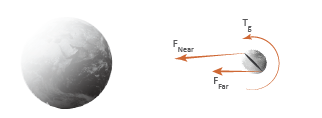You can model such torques in Simscape Multibody by modeling the different gravitational forces acting on a body. You do this using the Inverse Square Law Force or Gravitational Field block. If you use the Inverse Square Law Force block, you must create additional frames in each body whose response to gravitational torque you want to model. You must then apply a gravitational force to each frame explicitly. The figure shows an example.

Torque on the moon due to dissimilar gravitational forces at the elongated ends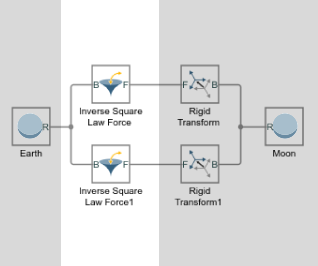If you use the Gravitational Field block, you must split each body into discrete sections and connect them through Weld Joint blocks. The Gravitational Field block automatically applies a force at the center of mass of each section, approximating the compound effect of the different gravitational forces on the body—which in this case is treated as a rigid multibody system. The figure shows an example.

Torque on the moon due to dissimilar gravitational forces at the elongated ends